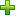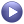# MV-3020: Optimize a Two Spring Mass System

In this tutorial you will learn how to setup an optimization problem using MotionSolve’s Optimization Wizard.

MotionSolve is commonly used for performing system level simulation. Simulations are commonly performed to understand how well a specific design performs. Often a goal for such simulations is to find the right set of design parameters that permit the system to perform its intended functions in some optimal way.

Commonly used design variables are the location and orientation of various connectors and their force characteristics. Occasionally the mass and material properties of some bodies are also included as design variables. The system behavior is normally characterized with a set of response variables. So, the goal of simulations often is to find the values of these design variables such that the response variables attain a desired set of values.

In the past such analysis has been done using techniques such as Monte Carlo simulations and design of experiments. These methods work quite well, but they are computationally intensive and require large sets of simulations.

MotionSolve now supports a capability for analytically computing design sensitivities. Design sensitivity is the matrix of partial derivatives of the response variables with respect to the design variables. A gradient-based optimizer is capable of using these sensitivities to minimize a cost function. This process is known as design optimization. A new optimization toolkit that permits optimization of some design problems is also now available in MotionSolve.

Though not as general as the statistical methods, optimization with design sensitivity is significantly faster and is the preferred solution in many instances.

In this tutorial you will learn about the following:
• Process of Optimization with MotionSolve
• Defining spring stiffnesses as design variables
• Defining displacements as responses
• Using the responses as objectives
• Running the optimization and post-processing the results
Introduction

Two springs are connected in series. The stiffness of spring-1 is k1 and that of spring-2 is k2. One end of spring-1 is fixed to ground while a force, P1, acts at the other end. Spring-2 is subjected to a force of P2 at the other end.

The objective of the analysis is to determine the sensitivities of stiffnesses of the springs to displacements u1 and u2 and use them to identify or tune k1 and k2 of the system to achieve specific values of u1 and u2.

MotionSolve's DSA (Design Sensitivity Analysis) capability to calculate sensitivities is utilized in this example. A Step-by-step procedure to define and run the model is given in the tutorial.

Figure 1 shows that problem setup. Properties used in the problem are also provided.
A list of properties for the system follows:
• Displacement: u0 =0 (Fixed)
• Force:
• P1 = 1N
• P2 = 2N
• Stiffness:
• k1 = 2 N/mm
• k2 = 3 N/mm
• Response Variables (RV): u1 and u2
• Design Variables (DV): k1 and k2
• Analysis Type: Static Analysis with DSA

The process of optimization starts with setting up a model in MotionView. MotionView’s Optimization Wizard is used to setup design variables, responses and objectives. The wizard also guides the user to run and plot/print results of optimization. It is also able to export a design from a particular iteration into a new MDL for further analyses.

In this step, you will add design variables for the optimization.

Before you begin, copy the file mv_3020_initial_two_springs.mdl located in the mbd_modeling\motionsolve\optimization\MV-3020 into your <working directory>.

1. Open mv_3020_initial_two_springs.mdl in MotionSolve.
2. In the Project Browser, right-click on Model and select Optimization Wizard from the context menu.
This will display the Optimization Wizard. The wizard consists of an Optimization Study pane that guides the user through each step of solving an optimization problem with MotionSolve. The wizard opens with the Design Variables page active that enables the selection of design variables.
3. Under Design Variables, click on the Springs tab.
Two SpringDampers will be listed:
• K1_SpringDamper
• K2_SpringDamper
The spring stiffness of these springs are made designable.
4. Expand the two springs by clicking on the ‘+’ button.
The data members that can be made designable are displayed as in Figure 3:
5. Using the Control keyboard button, select the k (stiffness) data members of both the springs. Click the Add button available at the bottom of the Model Tree.
The stiffness of SpringDampers are added as design variables with default upper and lower bound. The default value used by MotionSolve for calculating bounds is 10% of nominal value.
6. Modify the upper and lower bounds for stiffness as shown in Table 1:
Table 1.
DV Lower Bound Upper Bound
sd_0.k 0.25 4.0
sd_1.k 0.25 4.0
Note: The number within parenthesis of the designable entities tab at the top (Springs(2) in this case) indicates the number of design variables defined of that entity type.
You have now finished defining design variables.

In this step, you will add response variables for the optimization.

There are two responses in this problem:
• Displacement u1: To make u1 to reach a value of 3, the metric (1-u1/3)**2 is used.
• Displacement u2: To make u2 to reach a value of 4, the metric (1-u2/4)**2 is used.

1. Click on the Responses page.
2. Clickto add a response variable. In the dialog that appears, change the Label to u1 and click OK.
The ResponseVariable is added and appears in the list. The panel also appears.
3. In the panel, use the combo box to change the Response Type to Generic.
4. Accept the default value of 1.0 for the scale.
5. In the Response Expression key, enter the following expression:

(1-DM({b_0.cm.id},{m_0.id})/3)**2

Where:

b_0.cm.id = ID of the CM marker of Body 0

m_0.id = ID of the marker on ground body located at u1_Point 1

Note: During optimization, the optimizer tries to minimize the entire expression and as the value of DM({b_0.cm.id},{m_0.id}) approaches 3, the value of this response is minimized.
6. Check on the Use derivative check box.
When checked, the computed value of the expression at the last time step of the simulation is used as the response.
7. Repeat the steps above to add another ResponseVariable u2 with the following specifications:
• Type = Generic
• Response Expression = (1- DM({b_1.cm.id},{m_1.id})/4)**2
You have now finished adding responses.

Now you will add two objectives to the problem.

The objectives are as follows: the value of u1 should be 3 and value of u2 should be 4 at the end of optimization. You can use the responses you created in the previous section as objectives.

1. Navigate to the Goals page.
2. Under Objectives, click thebutton.
This will add an objective with the response rv_u1.
3. Change the Weight to 1000. Retain the Type as Min in order to minimize the response.
4. Repeat steps 2 and 3 to add a second objective (rv_u2).
There are no constraints in this problem. You have completed model setup.

## Run the Optimization

In this step you will run the optimization.

1. Navigate to the Solutions page to specify optimization settings and run the analysis.
Note: The model is saved before running, and if this is not desired, the model can be saved with a different mdl file name before starting the optimization. This can be done by closing the wizard, saving the model with a different name and returning to the wizard again.
2. Click on the Optimization Settings button. In the Optimizer Settings dialog that appears, change Accuracy to 1.0e-5.
3. Retain the rest of the parameters with default values. Close the dialog.
4. Click on Save & Optimize to start the optimization.
While the optimization is running, a plot of total weighted cost vs. iteration number and a plot of individual cost vs. iteration number are displayed in a separate window (after the initial simulation).

Once the optimization is complete a summary is listed in the text window.

Note: You may have to scroll down to the end and/or to the right to see results of the optimization.
The text window should appear as in Figure 8:
The following list shows important information available in the output window:
• Optimizer settings
• Iteration summary - Value of cost function by iteration number
• Results from optimization - Initial cost, final cost and percentage reduction in cost
• Final value of each objective at the end of optimization
• Final design table with information on design variable bounds and initial and optimized values of design variables
• Elapsed time for calculating cost and sensitivity

## Post-Process

In this step, you will post-process the results of optimization.

MotionView provides the ability to list, plot and animate results of optimization. It also provides capability to export mdl model corresponding to any design iteration.

1. Navigate to the Review Results page.
The Summary tab appears that lists the history of design variables, responses and objective functions in a tabular format for each iteration of optimization run.
Note: MotionSolve uses SLSQP algorithm for optimization. Hence the last iteration is usually the optimum configuration with least value of cost function. See Figure 9.
2. Click the Plot tab.
It helps to visualize a variation of design variables, response variables and cost function using graphs. You can choose to plot any number of variables along the y-axis with respect to a variable along the x-axis.
3. Select Iteration from the X list and Overall Objective from the Y list. Then click Apply.
Note: You can select and plot multiple items from the Y list.
4. Select the Animation tab to animate the configuration generated during any iteration.
5. Load the results file from the last iteration (iteration 16).
1. In the Iteration drop-down menu, choose 16.
3. Click(Play).